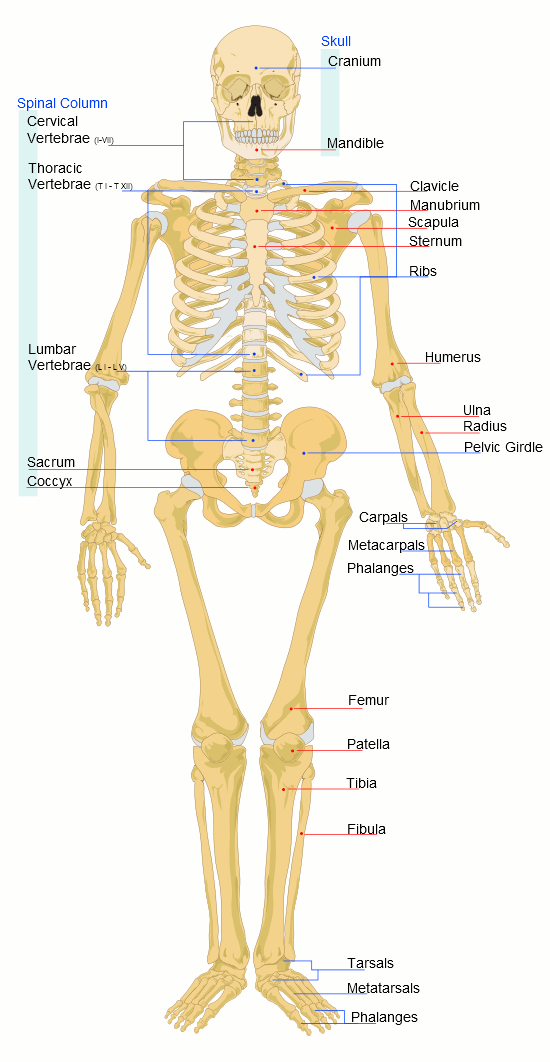# Substitute Into Expressions

Substitution means to work out an algebraic expression or equation by placing a number instead of a variable (letter).

A common example that you have done is to substitute numbers into formulae such as perimeter and area rules.

## Example One - Area of Rectangle

A rectangle has a length of 10 cm and a width of 4 cm. What is its area?

Area of Rectangle
= L × W
= 10 × 4
= 40 cm2

## Example Two - Forensic ScienceForensic scientists have found the human height can be estimated using equations involving the lengths of various bones. One such equation is:

Height in cm = Femur length in cm × 2.6 + 65

where the femur is the upper leg bone. If a femur bone is 40 cm long, what is the estimated height of the person?

Height
= 40 × 2.6 + 65
= 169 cm

## Example Three - Stopping Distance of a Car

When new roads are constructed, civil engineers calculate the maximum safe speed limits, particularly on dangerous bends. The formulae used to calculate a car's stopping distance is:

 Distance = (210 + 97 × R) × S 1000

where D = Distance (metres), R = Road Index and S = Speed (in km/h). What is the stopping distance of a car travelling at 80 km/h on a dry asphalt road (Road index of 1.3)?

 Distance = (210 + 97 × 1.3) × 80 = 26.9 metres 1000

## Maths Fun - Car Crash

Police use the Stopping Distance equation above to calculate the speed of a car when a fatality has occurred. What is the speed of a car on a dry asphalt road where the skid marks are 50 metres long? (Hint: Work backwards.)

## Questions

Find the value of the expression ab + cd + 3bd where:

Q1. a = 1, b = 2, c = 3 and d = 4
Q2. a = –2, b = –4, c = 6 and d = 12